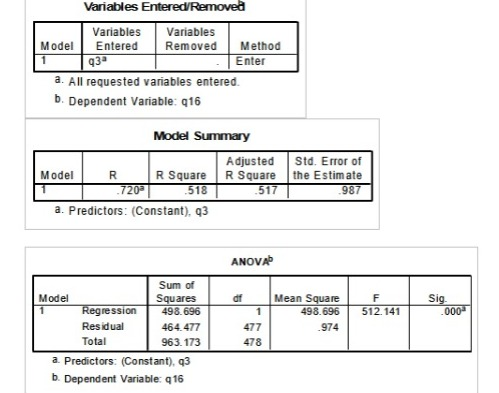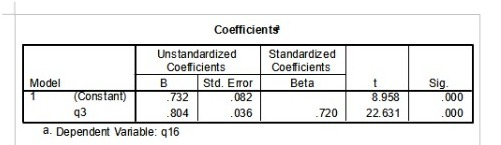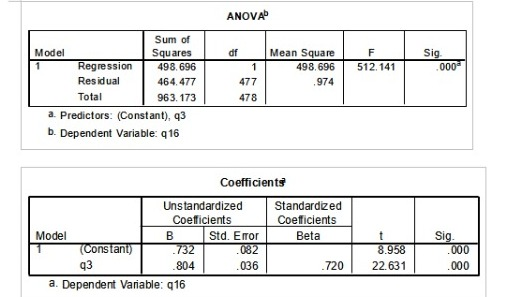# SPSS Data Analysis Homework Solution Sample

QUESTION

1. A correlation analysis was conducted with 10 survey questions from the Schwarzenegger survey (q1 through q10), producing the following results:

Descriptive Statistics

 Mean Std. Deviation N q1 2.66 1.529 496 q30 1.44 1.034 473 q32 1.95 1.372 470 q47 1.38 4.754 462 q52 4.04 .932 464 q53 2.06 .785 461

Correlations

 q1 q30 q32 q47 q52 q53 q1 Pearson Correlation 1 .492(**) .354(**) .127(**) -.067 -.531(**) Sig. (2-tailed) .000 .000 .006 .153 .000 N 496 471 468 461 462 459 q30 Pearson Correlation .492(**) 1 .530(**) .281(**) -.176(**) -.217(**) Sig. (2-tailed) .000 .000 .000 .000 .000 N 471 473 463 450 453 449 q32 Pearson Correlation .354(**) .530(**) 1 .186(**) -.126(**) -.112(*) Sig. (2-tailed) .000 .000 .000 .007 .017 N 468 463 470 450 454 450 q47 Pearson Correlation .127(**) .281(**) .186(**) 1 -.111(*) -.061 Sig. (2-tailed) .006 .000 .000 .019 .196 N 461 450 450 462 447 445 q52 Pearson Correlation -.067 -.176(**) -.126(**) -.111(*) 1 .036 Sig. (2-tailed) .153 .000 .007 .019 .447 N 462 453 454 447 464 457 q53 Pearson Correlation -.531(**) -.217(**) -.112(*) -.061 .036 1 Sig. (2-tailed) .000 .000 .017 .196 .447 N 459 449 450 445 457 461

** Correlation is significant at the 0.01 level (2-tailed).

* Correlation is significant at the 0.05 level (2-tailed).

1. Questions to answer (5 pts)

1. What two variables have the smallest linear association?

1. What is the relationship between q47 and q52?

1. Is the correlation between q1 and q52 significant?

1. What is the strongest association?

1. Is the sample size adequate to test hypotheses with these survey items?

2. A simple regression analysis produced the following results:

Descriptive Statistics

 Mean Std. Deviation N q16 2.27 1.420 479 q3 1.92 1.270 479

Correlations

 q16 q3 Pearson Correlation q16 1.000 .720 q3 .720 1.000 Sig. (1-tailed) q16 . .000 q3 .000 . N q16 479 479 q3 479 4791. Questions to answer (5 pts)

1. Is this regression model significant? Which statistic indicates this?

1. Is the amount of variance explained by this regression model larger or smaller than the unexplained variance? How do you know?

1. Does this result support the following hypothesis? How do you know?

H1: Modeling the behavior of Schwarzenegger (q3) will be positively associated with

increasing personal fitness habits (q16).

1. Is q3 a significant predictor of q16? How do you know?

1. Please write the unstandardized regression equation for this analysis below

1. A correlation analysis was conducted with 10 survey questions from the Schwarzenegger survey (q1 through q10), producing the following results:

Descriptive Statistics

 Mean Std. Deviation N q1 2.66 1.529 496 q30 1.44 1.034 473 q32 1.95 1.372 470 q47 1.38 4.754 462 q52 4.04 .932 464 q53 2.06 .785 461

Correlations

 q1 q30 q32 q47 q52 q53 q1 Pearson Correlation 1 .492(**) .354(**) .127(**) -.067 -.531(**) Sig. (2-tailed) .000 .000 .006 .153 .000 N 496 471 468 461 462 459 q30 Pearson Correlation .492(**) 1 .530(**) .281(**) -.176(**) -.217(**) Sig. (2-tailed) .000 .000 .000 .000 .000 N 471 473 463 450 453 449 q32 Pearson Correlation .354(**) .530(**) 1 .186(**) -.126(**) -.112(*) Sig. (2-tailed) .000 .000 .000 .007 .017 N 468 463 470 450 454 450 q47 Pearson Correlation .127(**) .281(**) .186(**) 1 -.111(*) -.061 Sig. (2-tailed) .006 .000 .000 .019 .196 N 461 450 450 462 447 445 q52 Pearson Correlation -.067 -.176(**) -.126(**) -.111(*) 1 .036 Sig. (2-tailed) .153 .000 .007 .019 .447 N 462 453 454 447 464 457 q53 Pearson Correlation -.531(**) -.217(**) -.112(*) -.061 .036 1 Sig. (2-tailed) .000 .000 .017 .196 .447 N 459 449 450 445 457 461

** Correlation is significant at the 0.01 level (2-tailed).

* Correlation is significant at the 0.05 level (2-tailed).

1. What two variables have the smallest linear association?

Ans. Q53 and q52 – Correlation value is .036

1. What is the relationship between q47 and q52?

Ans. Correlation value is -0.111 which is significant at 5%. It means that they have weak negative linear correlation.

1. Is the correlation between q1 and q52 significant?

Ans. No, since the sig. value is very high.

1. What is the strongest association?

Ans. Strongest correlation is between q1 and q53, also it is highly significant.

1. Is the sample size adequate to test hypotheses with these survey items?

Ans. Yes, any number greater than 30 is adequate.

2. A simple regression analysis produced the following results:

Descriptive Statistics

 Mean Std. Deviation N q16 2.27 1.420 479 q3 1.92 1.270 479

Correlations

 q16 q3 Pearson Correlation q16 1.000 .720 q3 .720 1.000 Sig. (1-tailed) q16 . .000 q3 .000 . N q16 479 479 q3 479 4791. Questions to answer (5 pts)

1. Is this regression model significant? Which statistic indicates this?

Ans. Yes, this model is significant. Sig. value in Anova table tells this.

1. Is the amount of variance explained by this regression model larger or smaller than the unexplained variance? How do you know?

Ans. Amount of variance explained is given by adjusted R square, which is just above 50%. Amount of variance explained is larger than unexplained.

1. Does this result support the following hypothesis? How do you know?

H1: Modeling the behavior of Schwarzenegger (q3) will be positively associated with

increasing personal fitness habits (q16).

Ans. Yes, because the coefficient of q3 is positive.

1. Is q3 a significant predictor of q16? How do you know?

Ans. Yes, as shown by the Sig. value in Coefficients table

1. Please write the unstandardized regression equation for this analysis below:

Q16 = 0.732 + 0.804*(q3)

Looking for Statistics Assignment Help. Whatsapp us at +16469488918 or chat with our chat representative showing on lower right corner or order from here. You can also take help from our Live Assignment helper for any exam or live assignment related assistance.